Скачать презентацию A Switch Criterion for Defined Contribution Pension Schemes

32b03630ec284dfc052644aaf555af14.ppt

• Количество слайдов: 28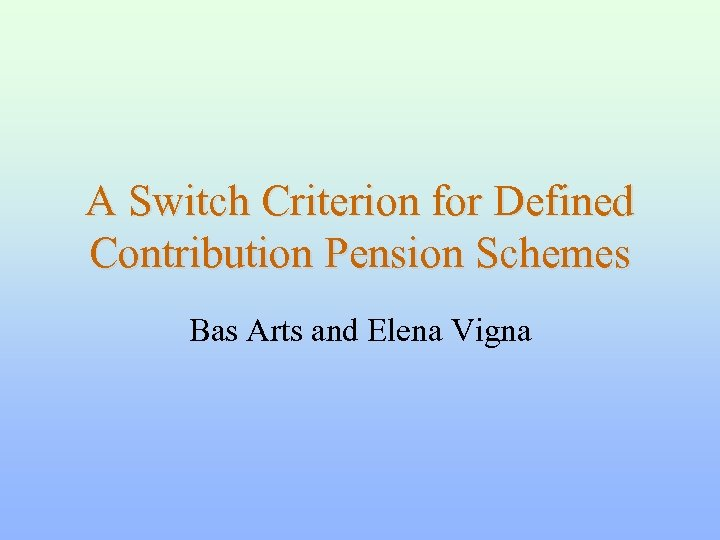A Switch Criterion for Defined Contribution Pension Schemes Bas Arts and Elena Vigna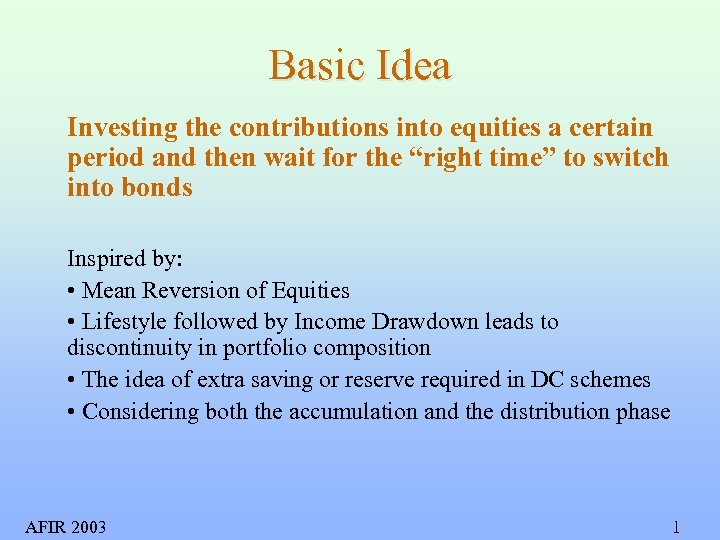Basic Idea Investing the contributions into equities a certain period and then wait for the “right time” to switch into bonds Inspired by: • Mean Reversion of Equities • Lifestyle followed by Income Drawdown leads to discontinuity in portfolio composition • The idea of extra saving or reserve required in DC schemes • Considering both the accumulation and the distribution phase AFIR 2003 1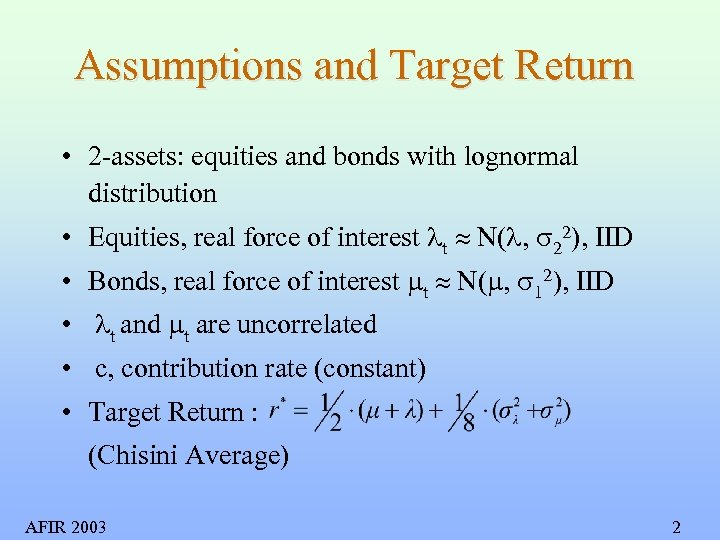Assumptions and Target Return • 2 -assets: equities and bonds with lognormal distribution • Equities, real force of interest t N( , 22), IID • Bonds, real force of interest t N( , 12), IID • t and t are uncorrelated • c, contribution rate (constant) • Target Return : (Chisini Average) AFIR 2003 2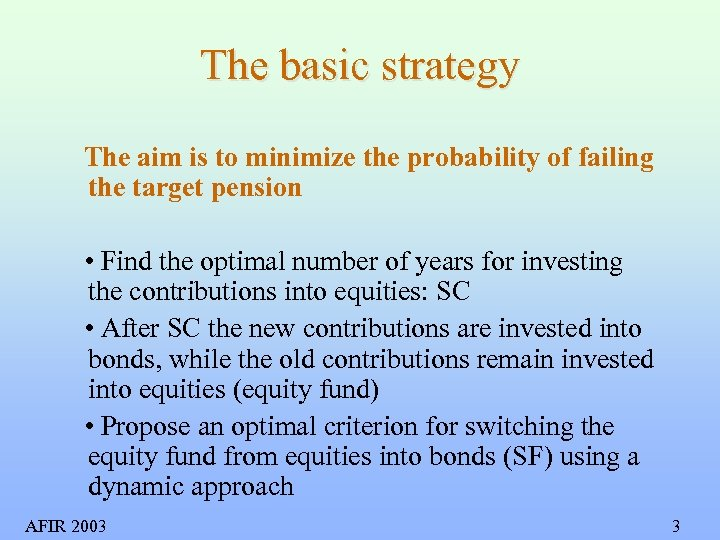The basic strategy The aim is to minimize the probability of failing the target pension • Find the optimal number of years for investing the contributions into equities: SC • After SC the new contributions are invested into bonds, while the old contributions remain invested into equities (equity fund) • Propose an optimal criterion for switching the equity fund from equities into bonds (SF) using a dynamic approach AFIR 2003 3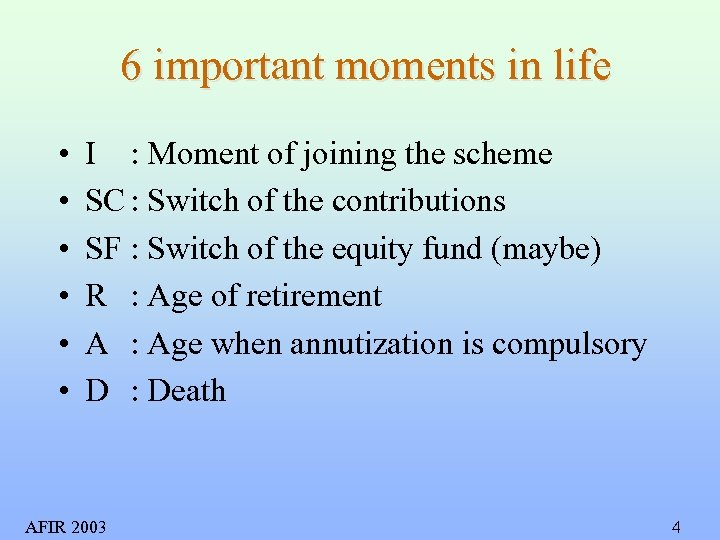6 important moments in life • • • I : Moment of joining the scheme SC : Switch of the contributions SF : Switch of the equity fund (maybe) R : Age of retirement A : Age when annutization is compulsory D : Death AFIR 2003 4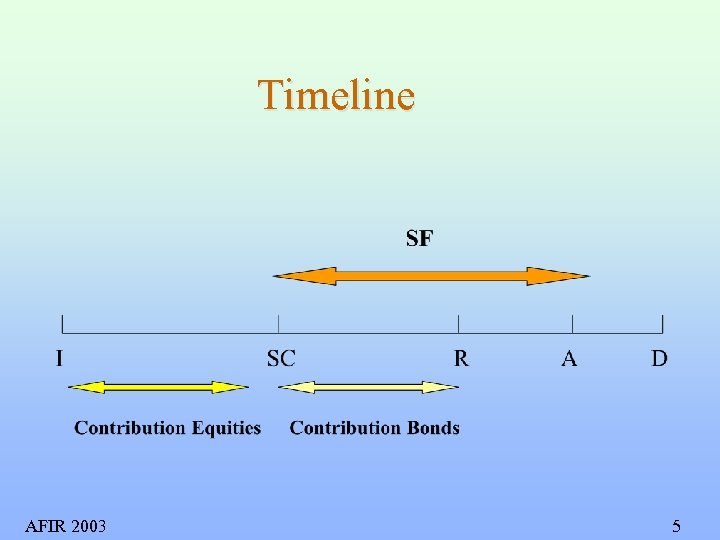Timeline AFIR 2003 5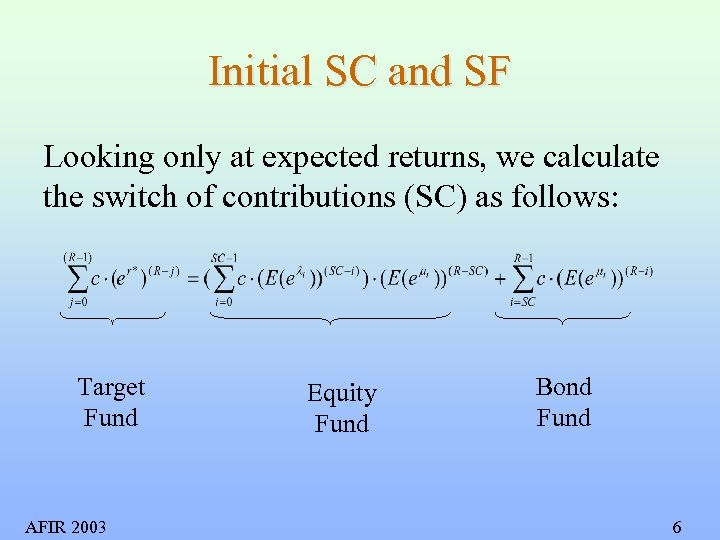Initial SC and SF Looking only at expected returns, we calculate the switch of contributions (SC) as follows: Target Fund AFIR 2003 Equity Fund Bond Fund 6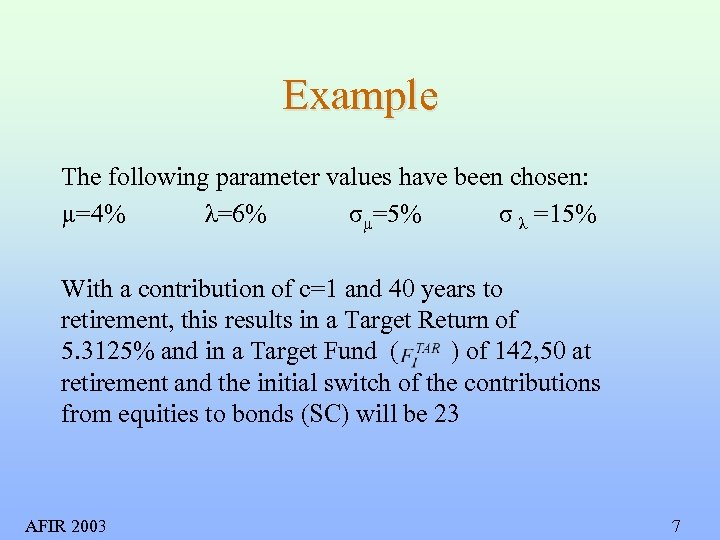Example The following parameter values have been chosen: µ=4% λ=6% σµ=5% σ λ =15% With a contribution of c=1 and 40 years to retirement, this results in a Target Return of 5. 3125% and in a Target Fund ( ) of 142, 50 at retirement and the initial switch of the contributions from equities to bonds (SC) will be 23 AFIR 2003 7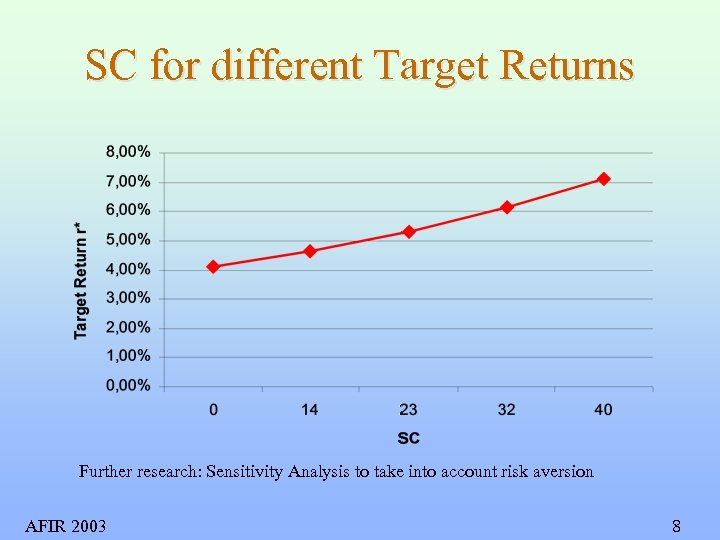SC for different Target Returns Further research: Sensitivity Analysis to take into account risk aversion AFIR 2003 8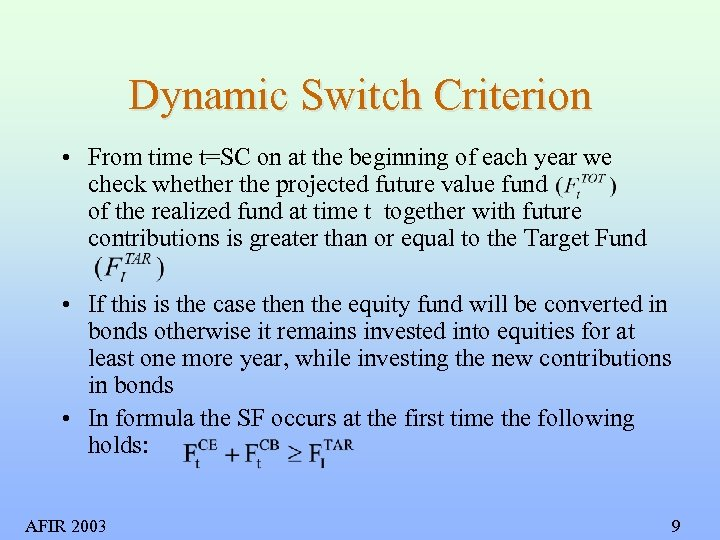Dynamic Switch Criterion • From time t=SC on at the beginning of each year we check whether the projected future value fund of the realized fund at time t together with future contributions is greater than or equal to the Target Fund • If this is the case then the equity fund will be converted in bonds otherwise it remains invested into equities for at least one more year, while investing the new contributions in bonds • In formula the SF occurs at the first time the following holds: AFIR 2003 9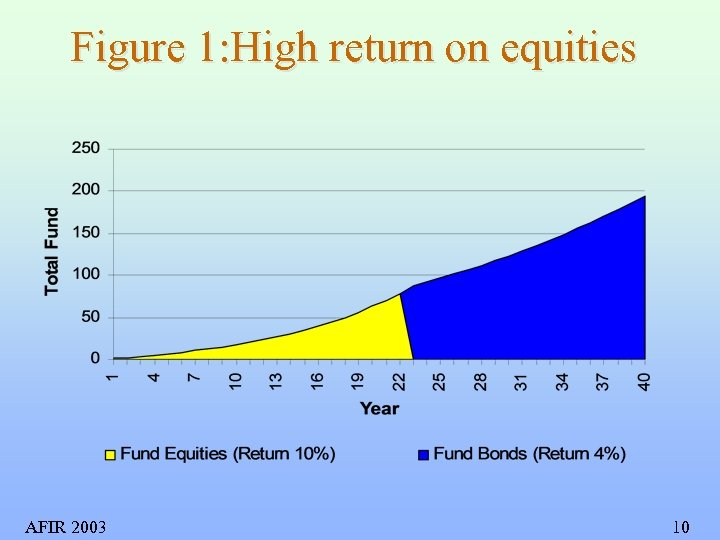Figure 1: High return on equities AFIR 2003 10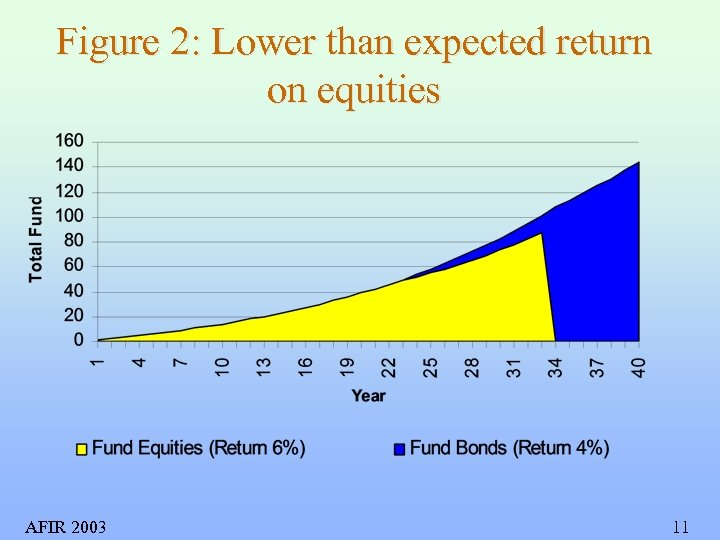Figure 2: Lower than expected return on equities AFIR 2003 11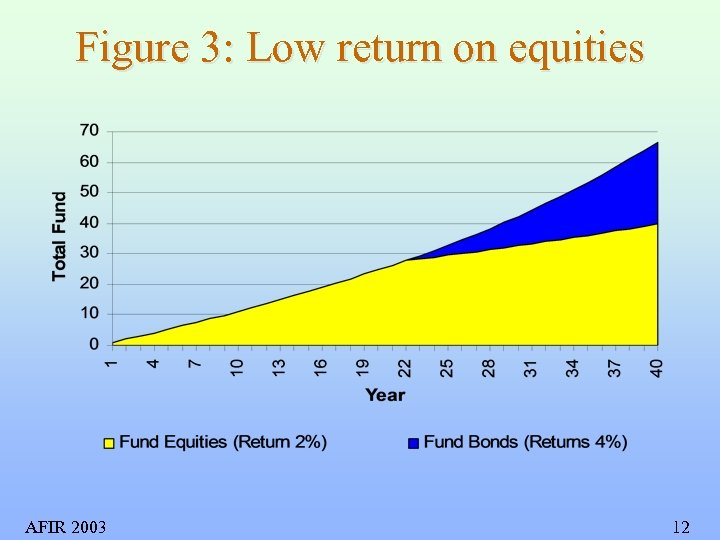Figure 3: Low return on equities AFIR 2003 12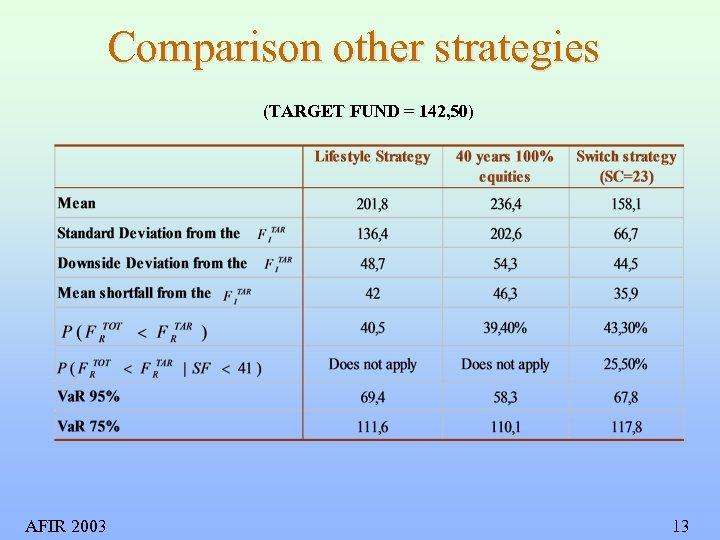Comparison other strategies (TARGET FUND = 142, 50) AFIR 2003 13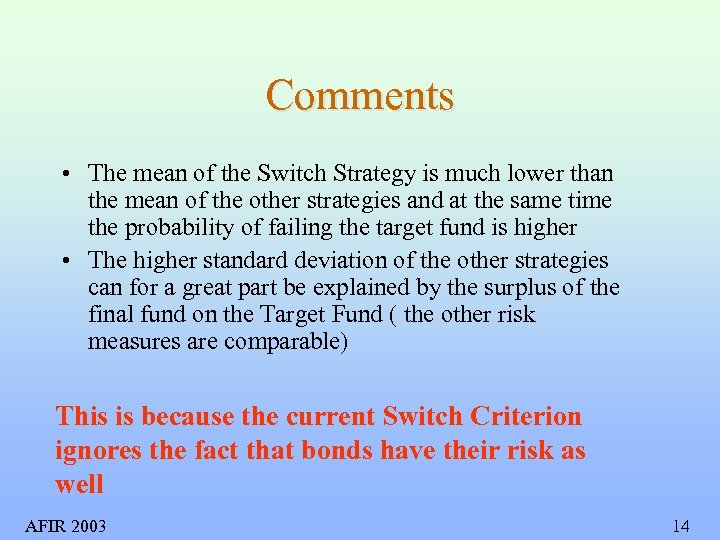Comments • The mean of the Switch Strategy is much lower than the mean of the other strategies and at the same time the probability of failing the target fund is higher • The higher standard deviation of the other strategies can for a great part be explained by the surplus of the final fund on the Target Fund ( the other risk measures are comparable) This is because the current Switch Criterion ignores the fact that bonds have their risk as well AFIR 2003 14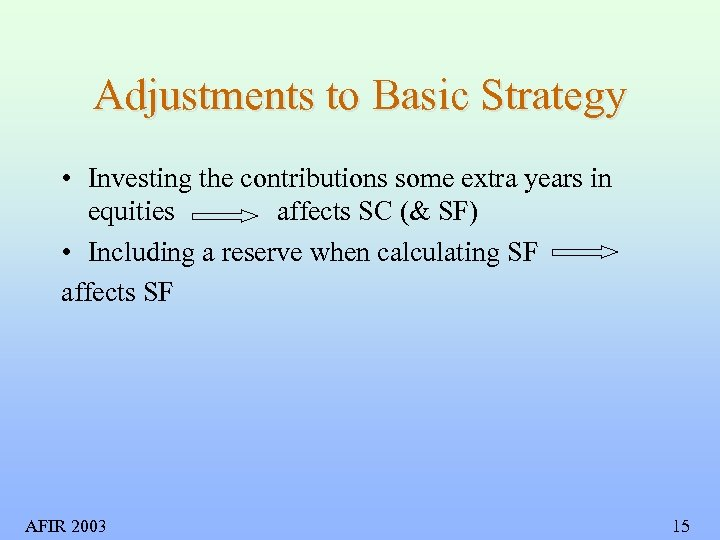Adjustments to Basic Strategy • Investing the contributions some extra years in equities affects SC (& SF) • Including a reserve when calculating SF affects SF AFIR 2003 15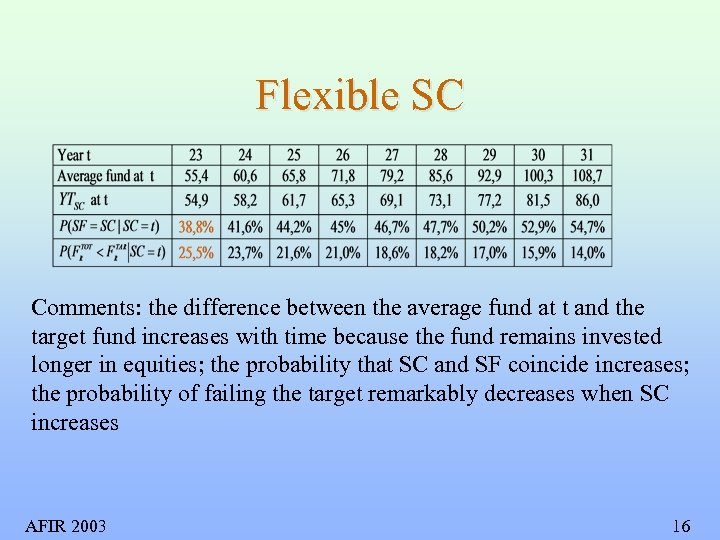Flexible SC Comments: the difference between the average fund at t and the target fund increases with time because the fund remains invested longer in equities; the probability that SC and SF coincide increases; the probability of failing the target remarkably decreases when SC increases AFIR 2003 16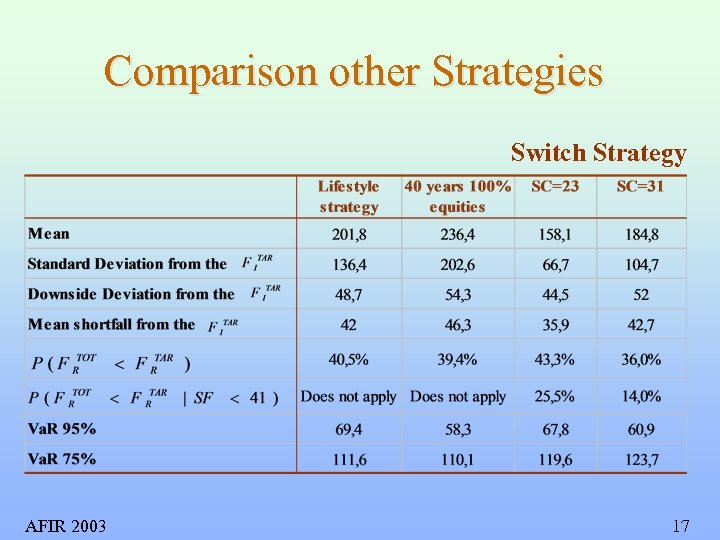Comparison other Strategies Switch Strategy AFIR 2003 17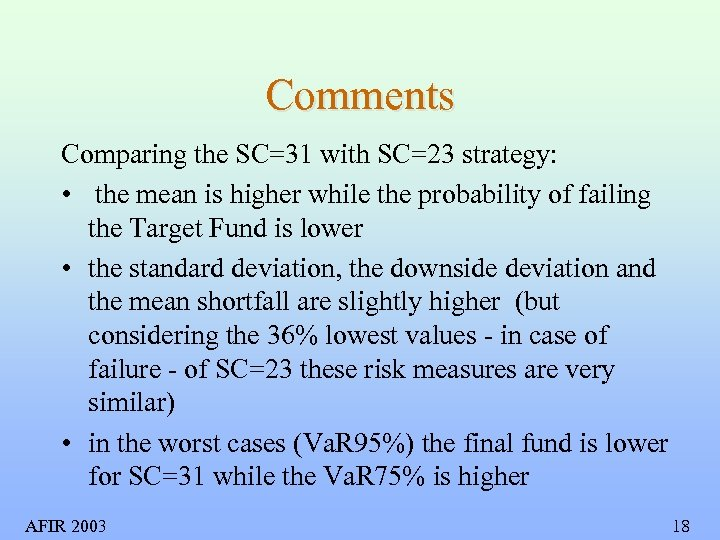Comments Comparing the SC=31 with SC=23 strategy: • the mean is higher while the probability of failing the Target Fund is lower • the standard deviation, the downside deviation and the mean shortfall are slightly higher (but considering the 36% lowest values - in case of failure - of SC=23 these risk measures are very similar) • in the worst cases (Va. R 95%) the final fund is lower for SC=31 while the Va. R 75% is higher AFIR 2003 18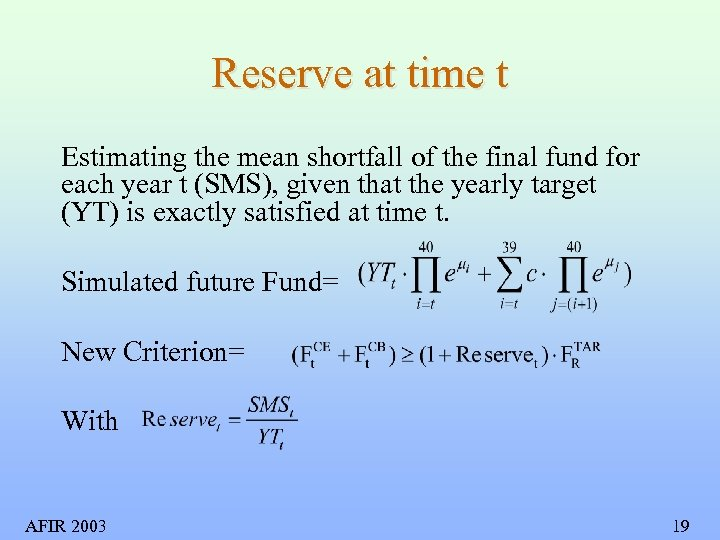Reserve at time t Estimating the mean shortfall of the final fund for each year t (SMS), given that the yearly target (YT) is exactly satisfied at time t. Simulated future Fund= New Criterion= With AFIR 2003 19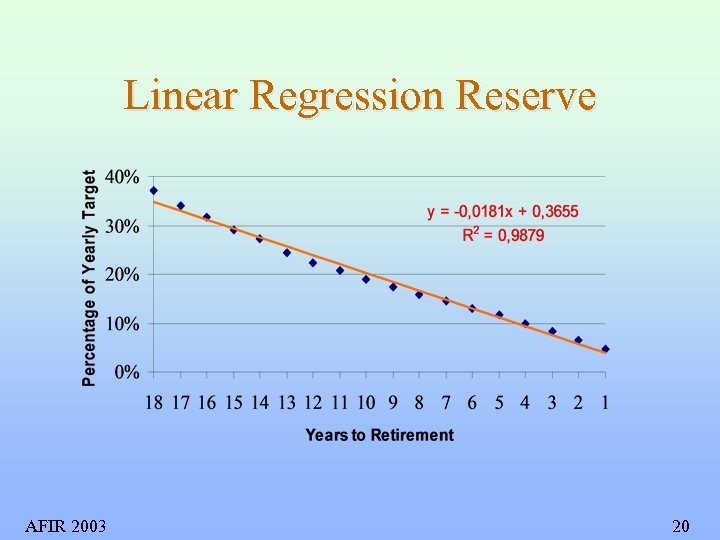Linear Regression Reserve AFIR 2003 20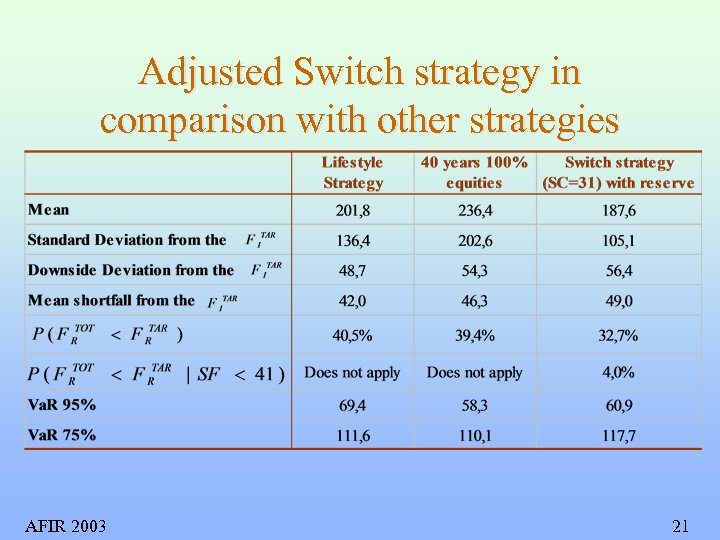Adjusted Switch strategy in comparison with other strategies AFIR 2003 21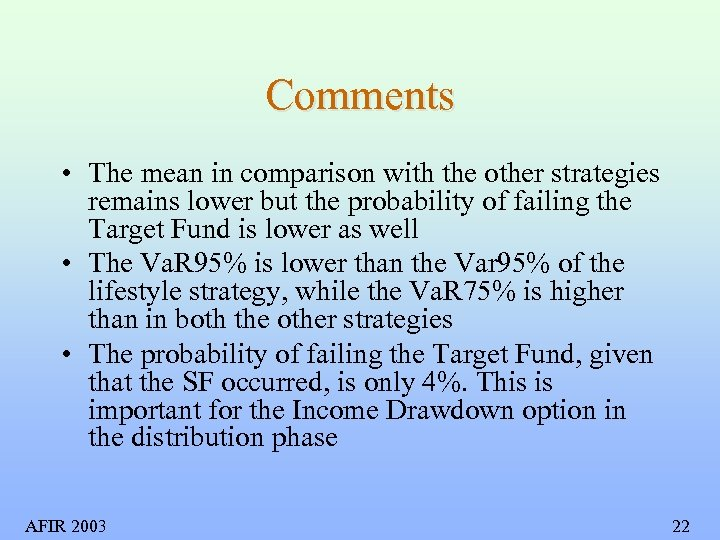Comments • The mean in comparison with the other strategies remains lower but the probability of failing the Target Fund is lower as well • The Va. R 95% is lower than the Var 95% of the lifestyle strategy, while the Va. R 75% is higher than in both the other strategies • The probability of failing the Target Fund, given that the SF occurred, is only 4%. This is important for the Income Drawdown option in the distribution phase AFIR 2003 22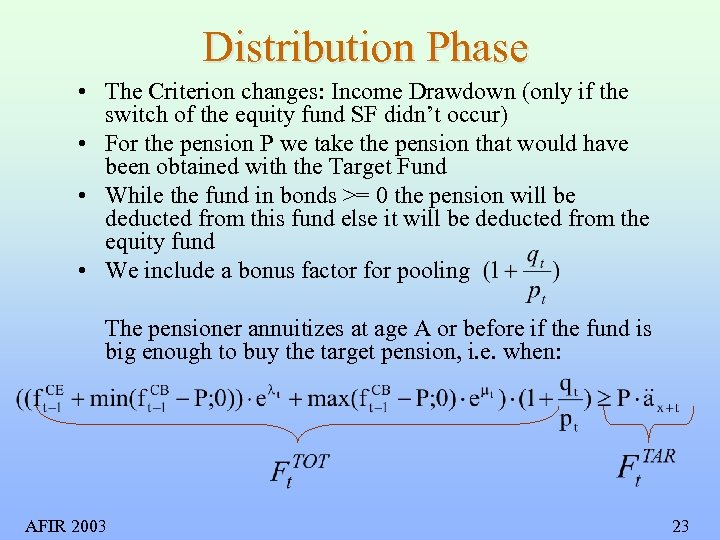Distribution Phase • The Criterion changes: Income Drawdown (only if the switch of the equity fund SF didn’t occur) • For the pension P we take the pension that would have been obtained with the Target Fund • While the fund in bonds >= 0 the pension will be deducted from this fund else it will be deducted from the equity fund • We include a bonus factor for pooling The pensioner annuitizes at age A or before if the fund is big enough to buy the target pension, i. e. when: AFIR 2003 23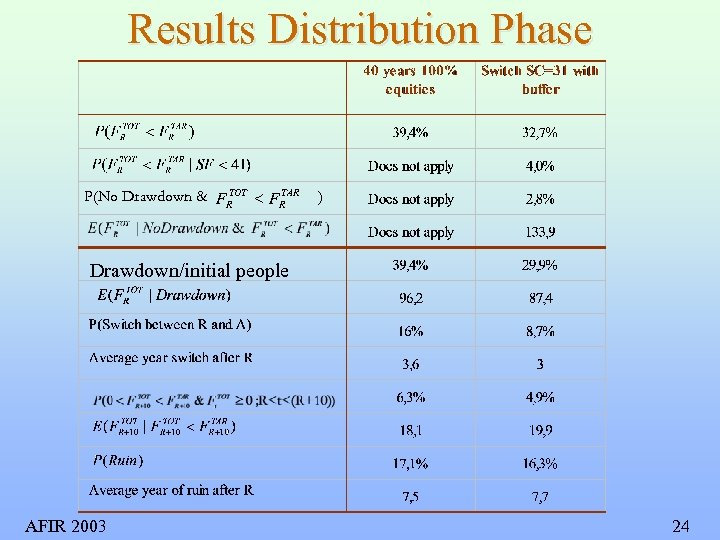Results Distribution Phase TOT TAR P(No Drawdown & FR < FR ) Drawdown/initial people AFIR 2003 24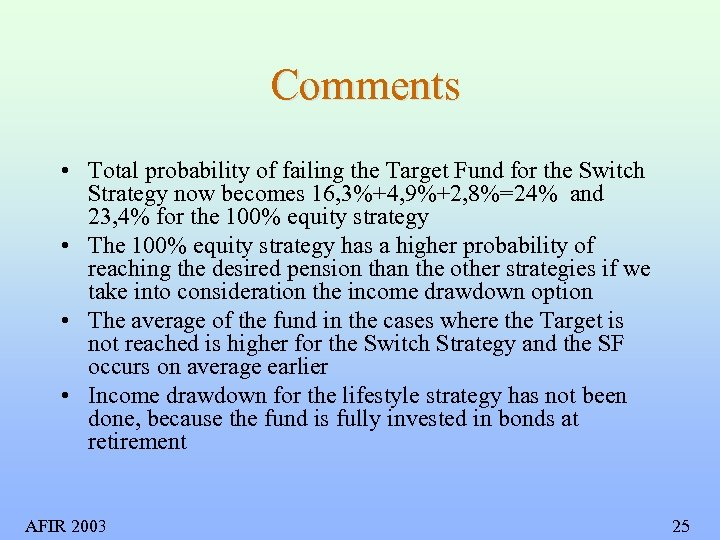Comments • Total probability of failing the Target Fund for the Switch Strategy now becomes 16, 3%+4, 9%+2, 8%=24% and 23, 4% for the 100% equity strategy • The 100% equity strategy has a higher probability of reaching the desired pension than the other strategies if we take into consideration the income drawdown option • The average of the fund in the cases where the Target is not reached is higher for the Switch Strategy and the SF occurs on average earlier • Income drawdown for the lifestyle strategy has not been done, because the fund is fully invested in bonds at retirement AFIR 2003 25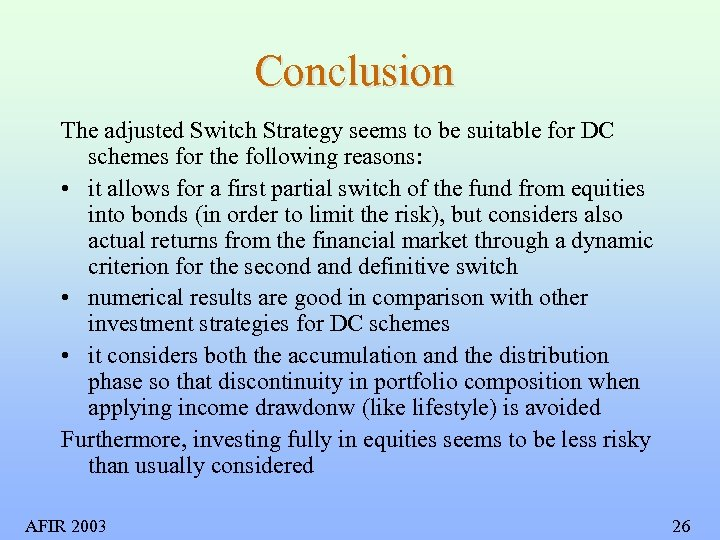Conclusion The adjusted Switch Strategy seems to be suitable for DC schemes for the following reasons: • it allows for a first partial switch of the fund from equities into bonds (in order to limit the risk), but considers also actual returns from the financial market through a dynamic criterion for the second and definitive switch • numerical results are good in comparison with other investment strategies for DC schemes • it considers both the accumulation and the distribution phase so that discontinuity in portfolio composition when applying income drawdonw (like lifestyle) is avoided Furthermore, investing fully in equities seems to be less risky than usually considered AFIR 2003 26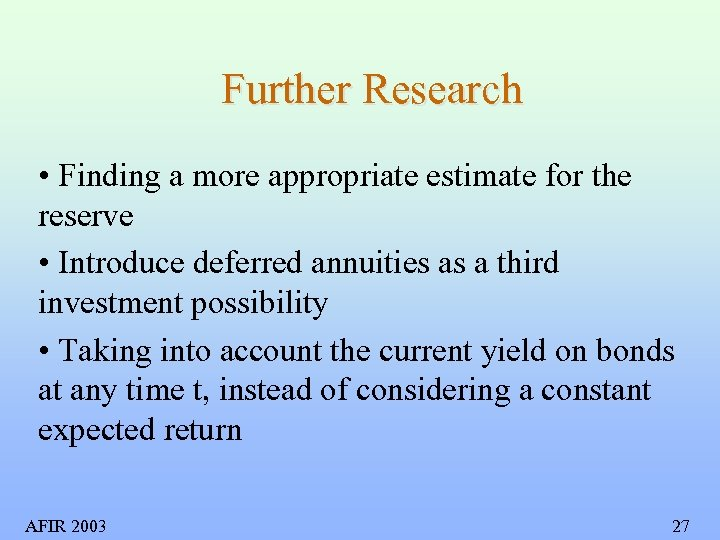Further Research • Finding a more appropriate estimate for the reserve • Introduce deferred annuities as a third investment possibility • Taking into account the current yield on bonds at any time t, instead of considering a constant expected return AFIR 2003 27